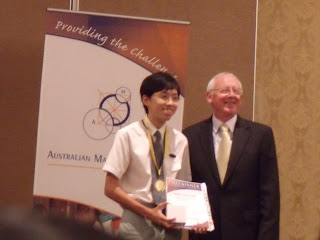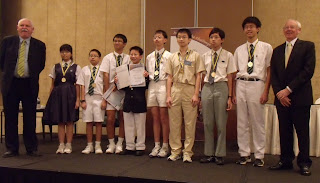Thursday, 27 October 2011

AMC Annual Awards Presentation 2011

The Australian Mathematics Competition (AMC) Annual Awards Presentation was held at the Novotel Clarke Quay Hotel this evening.The medal winners: from left to right (Junior) Song Somin, Liu Tianyi, Liu Yijia, Sheldon Tan, Xavier Lim, (Intermediate) Zeng Yi, Lim Jeck, (Senior) Ding Yue.Tuesday, 18 October 2011

Playing Piano

This is posted by LL ™ (press ctrl and 0153 to get the ™)

Hi. This is the 2nd song I learnt. It is called Hokage's Funeral from Naruto. I am in piano grade zero because I have never learnt piano from any teacher. There were some mistakes I made, but I was too lazy to retake after so many NGs. Other songs that I have learnt to play include: Itsumo Nando Demo from Spirited Away, Sadness and Sorrow from Naruto, Super Mario Brothers: Underwater Theme, 月亮代表我的心 and Tsuna Awakens from Hitman Reborn.

Tuesday, 11 October 2011

Mistakes in Olympiad Maths Trainer 6 Book

This is posted by Lim Li ☺☻My mother bought this book from Popular Bookstore for me to practise last year. I found many mistakes. Now that I am so free (PSLE is over!), I will type out some of the mistakes. My comments are in Red.

Week 2 Qn 5.
(a) Choose any three letters from a, b, c and d. In how many ways can we arrange these letters?
(b) A teacher wants to choose a captain and a vice-captain among 12 volleyball players. In how many ways can he do so?
Answer: (a) 4A3 = 4 x 3 x 2 = 24
(b)12C2 = (12 x 11 )/(2 x 1 ) = 66
(a) Should be 4P3
(b) Captain and vice-captain is different person, should be 12P2

Week 5 Qn 2. In a mathematics quiz, Fredric answered 1/9 of the questions wrongly. Paul answered 7 questions correctly. The number of questions that both of them answered correctly was 1/6 of the total number of questions. How many questions were there in the quiz?
First of all, from the 3rd statement, 1/6 of the total number of questions, one would naturally assume that it is the total number of questions in the mathematics quiz rather than the total number of questions both answered together, which is twice the amount. We also have to assume that all questions are answered correctly or wrongly. Logical interpretation of the question would lead to 36 as the answer.

Week 5 Qn 5. In the figure below, how many triangles can be formed using any three points as the vertice?
. . .
. . .
. . .
The correct solution is 9C3=84, 84-8=76 Answer: 76
Week 6 Qn 3: For 1^2 + 2^2 + 3^2 + ... + n^2, we can compute n(n + 1) (n + 2) /6. Find the value of 1^2 + 2^2 + 3^2 + ... + 15.^2
The formula in the question is wrong. It should be n (n + 1) (2n + 1) /6
Week 7 Qn 1. Don and Andy have some marbles. If Don gives some marbles to Andy, the number of marbles that Don has is twice the number of marbles what Andy has. If Andy gives the same number of marbles to Don, the number of marbles that Don has is 4 times of what Andy has. How many marbles does each of them have at first?
If the question does not provide any number, how are we supposed to find the number of marbles each of them has? I think the question should be: "What is the least possible number of marbles each of them has at first?"
Week 10 Qn 6. An athlete ran up the staircase of a building as part of his training programme. If he ran up the building at first and then walked up the last 24 steps, it would take him 28 seconds. If he ran up the building at first and then walked the last 36 steps, it would take him 30 seconds. How many steps were there on the staircases?
It is an impossible question, which cannot be solved. Too little information is given. Assuming speed is integer, there will be 3 solutions, 72, 288, 720 steps. If speed is not integer, the number of steps can be any integer above something, 36 I think. Anyway, if you see the solution given, the first statement totally didn't make any sense, and the 2nd statement is even worse.

Other questions with mistakes that I am too lazy to type. Sorry, only people who have purchased the book will understand what I am typing.

Week 7 Qn 2:either the question should be unshaded or the answer should add a 200-85=115

Week 9 Qn 2: should write "only" for the 2 subjects e.g. 6 students like both mathematics and English only, instead of, 6 students liked both mathematics and English.

Week 10 Qn 1: the question says the number formed by the first 5 digits and the number formed by the last 3 digits is 68427 but the answer says 68247.

Week 13 Qn 5a: it says + but should be x

Week 14 Qn 2: firstly, the answer says 320/(64/48)=20. It should be 320/(64-48)=20. Secondly, you are assuming there is the same amount of chairs and tables, which is not stated in the question.

There are (a lot) more questions with mistakes, which I am even lazier to type.

Tuesday, 4 October 2011

PSLE Over!!!

This is posted by LL☺☻

PSLE is over!!!!( ! x a very big number) Now I can start to recycle all the stuff (no burning as it causes global warming). However, there is still HCL for those taking HCL, too bad for you :P.

How do I find each paper? Why am I asking myself? Because I am weird :)
[*very easy, ** easy, *** ok, **** hard, ***** very hard]
English [***] - easier than my school prelim exam but my English is VERY poor so I obviously did badly even if the paper is not difficult :(
Math [*] - too easy, I managed to check more than 3 times but unfortunately I did not spot a careless mistake in one of the questions - Paper 1 reading book question, I put 26 days when the correct answer should be 24 days :(
Chinese [***] - my teacher said it was considered a difficult paper as some questions were rather tricky :( I think I have made a few mistakes, they are not careless mistakes as I really am dumb
Science [**.5] - I completed the paper in about 1 hr 5 mins and have 40 minutes to check. As expected, I managed to find a few mistakes (since I am such a careless person) during checking.

Things to do after the PSLE:
1. hibernate
2. sleep
3. eat
4. sleep more
5. laugh at people who take HCL
6. play
7. tell everyone that 1+1=2
8. (same as 1)
9. do random things
10. go US before I'm 13 years old (note that US is not united states, hint: it is in Sentosa)